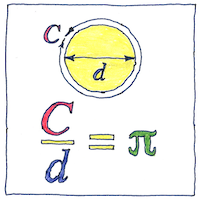# 1768 Pi

## Pi

The history of π (the ratio of the circumference to the diameter of a circle) is old. The Egyptians knew of it; the Babylonians knew of it; the Greek mathematician Archimedes used a polygon of ninety-six sides to calculate it; the Chinese mathematician Liu Hui knew of it and used polygons of one hundred ninety-two sides and three thousand seventy-two sides, calculating their areas rather than perimeters. Over the centuries, new methods for calculating π have resulted in increasing accuracy— but all decimal representations are approximations of a number that is never ending and never repeating.

## Proofs

In 1768, Johann Heinrich Lambert proved that π is irrational it cannot be expressed as a ratio of whole numbers and cannot have a repeating pattern. In 1882, Ferdinand von Lindemann proved that π is transcendental it cannot be a root of a polynomial equation with rational coefficients. One hopes, by now, inquiring minds have turned to problems that can be solved.

## Squaring the circle

The eye encompasses the obvious only and often is mistaken. Then the mind follows, convinced it should believe what it has seen. It doesn’t matter how hard we try to turn a base metal into gold to build a perpetual motion machine or to square the circle, human effort cannot controvert the intricate and subtle nature of our physical universe. Seldom, if ever, do we exactly hit the mark.

To “square the circle” is to find the length of the sides of a square with the same area as a circle of a given radius. The area of a circle of radius ``` r ``` is ``` πr2 ``` , so success depends on the nature of π. The proofs of Lambert and Lindemann mean that we know that it is impossible for anyone to construct on a flat surface a square with the same area as a given circle using a compass and ruler.

The expression “squaring the circle” means trying to do the impossible.

Archimedes used the “method of exhaustion” to approximate the value of π. The work of both Archimedes and Liu Hui prefigured calculus; Archimedes wrote of infinitesimals and Liu Hui described the use of the limit.